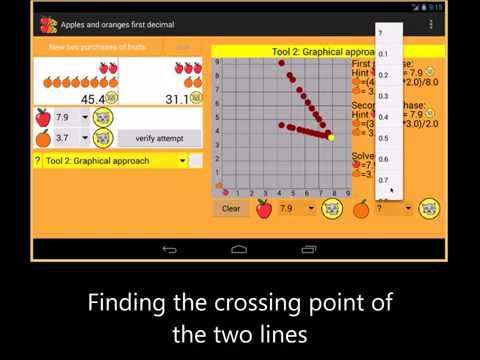# Apples and oranges 1 decimal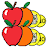10+Everyone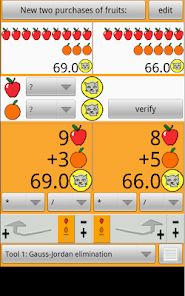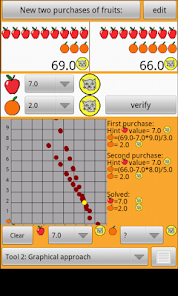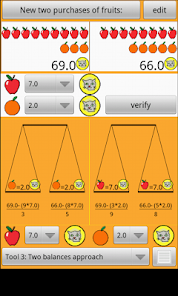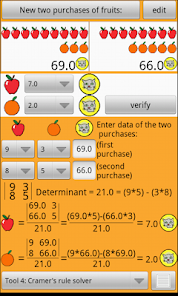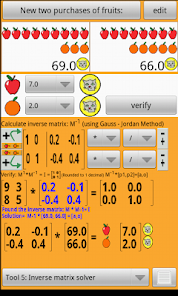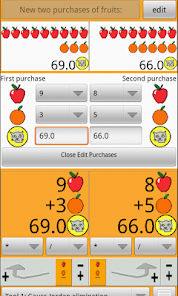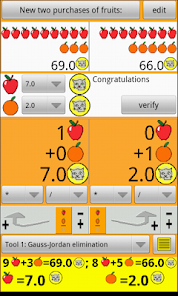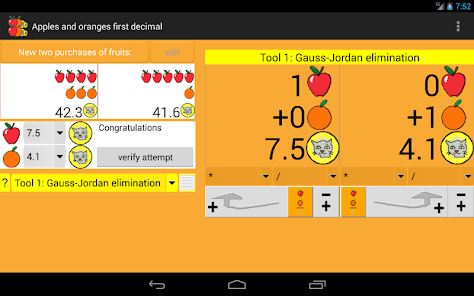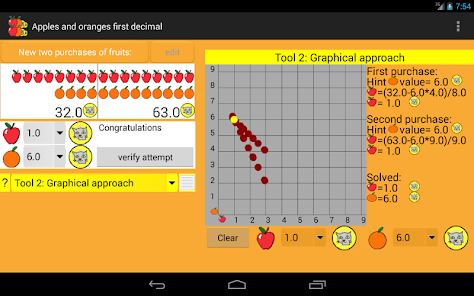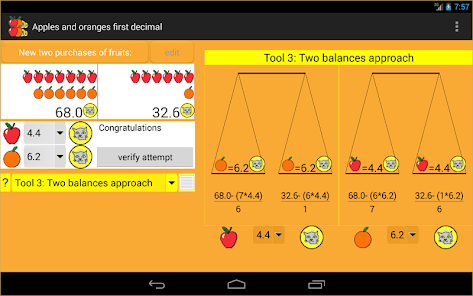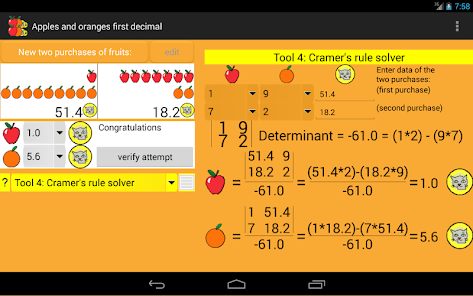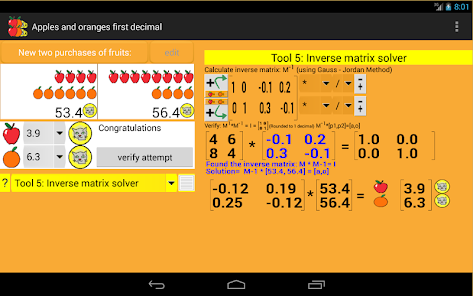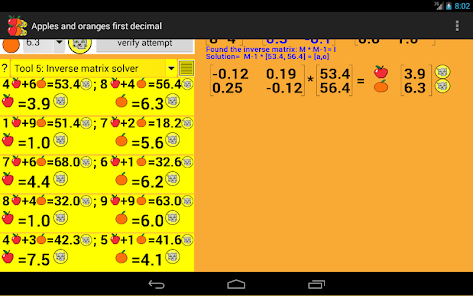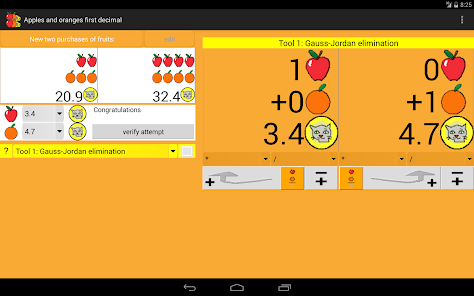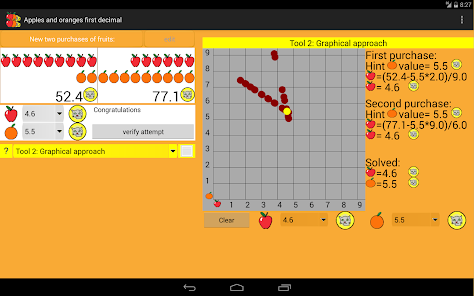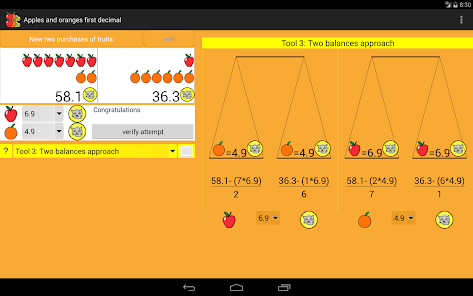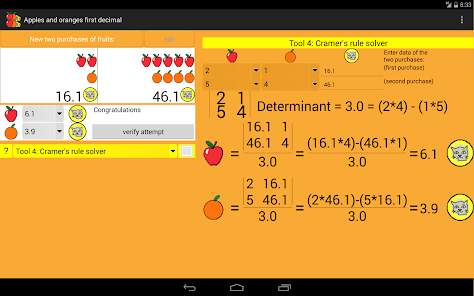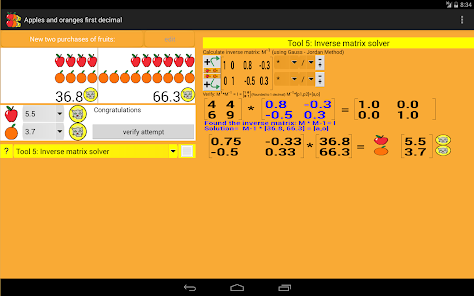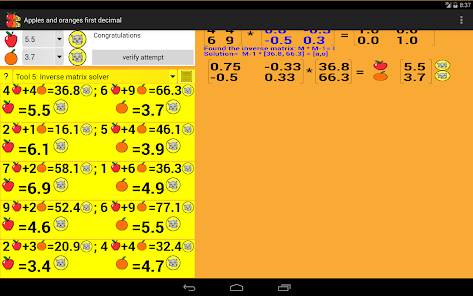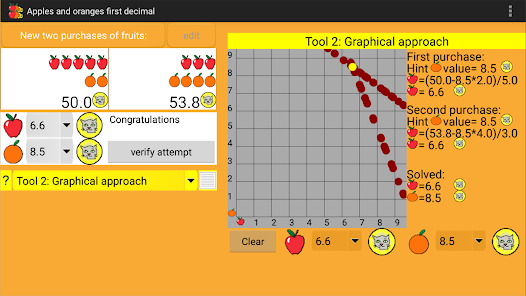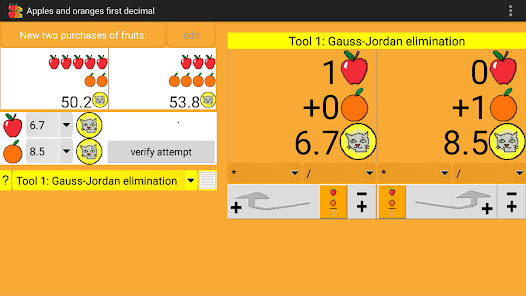Apples & Oranges - Algebra
Adding Apples and Oranges Version with 1 decimal

This new application is a basic tool to learn algebra.
Trying solving a system of linear equations with two equations and two unknown variables. (Two purchases)
Based on the same problem seen at the free "Adding Apples and Oranges." (https://play.google.com/store/apps/details?id=com.appleorange.addingapplesandoranges) where only used integers.
Now, this new program works externally with a decimal.

It contains:

. A button that generates new questions ("two new purchases of fruits").
. Two drop-downs to select a solution and a button to verify: The verification button checks the solution, and adds it to the list of the session.

You can edit the statements. The program checks if the determinant of the proposed statement is nonzero.

. The 5 tools to help resolve the problem:

* Tool 1: Gauss-Jordan elimination:

The two equations are represented as two purchases of fruits.
Contains tools to add one column to another, multiply, divide and change the sign for to get to have one apple on a side and and an orange at the other.
The result of the two additions are the values of apple and orange.

* Tool 2: Graphic:

Contains a graphic frame and 2 drop-downs to find the solution, using the method essay / error.
The problem posed is a linear system of equations, so the results must be ever into two lines that intersect.
To get the answer, one should find this point.

You can also click directly on the chart.
The graph is divided by the diagonal.
Below the diagonal, apple values are entered, for to get the values of the orange.
Above the diagonal, one enters the price of the orange.
The meeting point of the two lines is represented by a yellow dot.

* Tool 3: Two weighing scales:

Shows two weighing balances. On the left, due to its drop-down, which determines the value proposed for apples: results gives the price of oranges in terms of the two equations.
The right hand scale gives the results from the proposed price for oranges.
When a scale is balanced, gives the results of both the price of the apples and oranges.

* Tool 4: Cramer's rule:

This tool solves the problem at once.
Contrary to what happens in other tools, here we have to manually enter the data of the problem. The two equations (two purchases) are represented in horizontal rows.
If you have not entered data, the tool warns that you can not solve the problem.
It can also warn when the determinant is zero:

The determinant is zero when the two lines that we saw in the graphical tool, are not crossing. This happens when they are parallel. And the lines are parallel when a purchase (equation) is linear function of the other. (eg 1 A + 2 O= n1 and 2 A + 4 O = n2)
The program automatically never generates such problems.

* Tool 5: Inverse matrix:

The tool represents the terms of the equation automatically and in landscape format (each purchase is a row)
The resolution tool there are the terms of the two equations on the left and the identity matrix at right.
The objective is to calculate the inverse matrix.
This is achieved by operating the rows, until the data from the equations (on the left) become the identity matrix.
At this time to the right we have the inverse matrix.
The multiplication of the results vector by the inverse matrix gives the solution.

This program shows rounded numbers.
Internally works with all decimals needed.
If you try to do these calculations with a calculator, you'll see that this program displays the friendly face of algebra.

Mathforum MathTools Reference:
http://mathforum.org/mathtools/tool/219536/

Math 7: Linear relationships, Find slope, Slope-intercept form, Equation of the line, Rect coordinate geometry, First quadrant, Geometry in the plane

Algebra II: Solving systems of equations, Cramer's rule, Matrices, Elimination, Substitution

Nummolt apps: "Not Montessori per se, but Montessori-like"

Credits:
Feature graphic by: http://photo-lgz.blogspot.com/
Updated on
Sep 7, 2022

## Data safety

Safety starts with understanding how developers collect and share your data. Data privacy and security practices may vary based on your use, region, and age. The developer provided this information and may update it over time.No data shared with third parties